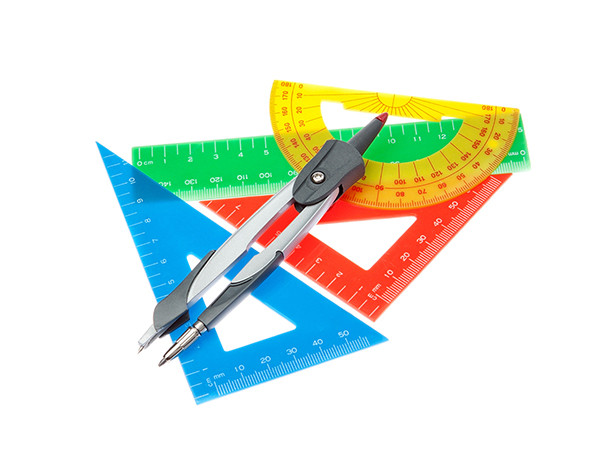# How much mathematics is like enough for college robotics?So how much of mathematics is needed for robotics? The question is subjective like all fields but here we will try to pick out the most common areas of mathematics which you would need to make an intermediate robot for your robotics club. This blog will talk purely on mathematics without much focus on electronics,physics and programming.

Linear algebra - This tops our list actually and you would need to brush up concepts on vectors, matrix, transpose, quadratic forms, determinants. You don't need to be a pro at linear algebra but doing a brush up of the core concepts will go miles when you deal with concepts related to kinematics, movements, computer vision.

Advanced Calculus - This one is no brainer right? You would need velocity which is a derivative of distance and you would need acceleration which is a derivative of velocity. Even motion is based on differential equations so why not make calculus your buddy?Geometry - Robots needs to work in 3 dimensions. You also would need to design your structure and how can one do these without geometry? Plane, joints,kinematic chain etc are needed for robotics.

Probability - Robots needs to do with uncertainty and act on certain conditions. You can not possibly ignore probability while making a decision making robot while you may skip it for basic ones.Applied mechanics - Well you can argue this to be physics but we still thought that we will keep applied mechanics in the list. You can not design an intermediate robot without knowing mechanics.

Now that you know the 5 wide areas of mathematics that are needed for a robotics club, the obvious question comes to mind is - what can be done if someone is weak or just not good in one or some areas? Well, we have 5 wide solutions as well for them. Read on.

Tip 1 # Make study notes of the core concepts and not just the formulas. You need to keep formulas in memory as implementation is done in programming and all languages these days have mathematical packages built for them. But don't compromise on the core concepts.

Tip2 # Read the section on Physical interpretation of the mathematics. Use the internet and Youtube for such interpretation. Tools like GNU Octave and Matlab can help you here as well.Tip3 # Try to teach someone.Yes, teaching someone is a good way to learn.

Tip4 # Ask why more than how. When you ask 'how' you are going to the solution mode first but if you ask 'why' you are giving yourself more chance to understand the problem. Let me give you an example - How do you do transpose? Answer - We revert the matrix to change the rows and columns to get a transpose. Right? Now ask - why do we need a transpose in this world? Aha - now you see the difference? There are multiple uses and one we can suggest to close this tip is to imagine you have stored a BW image as pixels in a matrix M.Now if you apply a transpose to this matrix M you would turn the image 90 degrees counter clockwise (vertical mirroring = MT).

Tip5 # Seek help. Ask some one who knows it. It can be a teacher, a friend or an internet forum. Get it clarified.

Hope you liked this blog and can focus more in your mathematics for that next super cool bot. Comment, share and like.